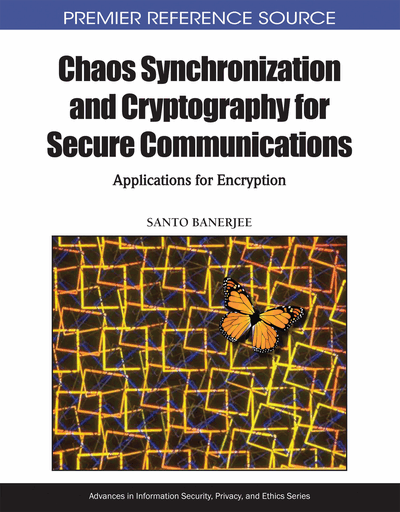# Synchronization in Integer and Fractional Order Chaotic Systems

Ahmed E. Matouk (Mansoura University, Egypt; Hail University, Saudi Arabia)
DOI: 10.4018/978-1-61520-737-4.ch007
Available
\$37.50
No Current Special Offers

## Abstract

In this chapter, the author introduces the basic methods of chaos synchronization in integer order systems, such as Pecora and Carroll method and One-Way coupling technique, applying these synchronization methods to the modified autonomous Duffing-Van der Pol system (MADVP). The conditional Lyapunov exponents (CLEs) are also calculated for the drive and response MADVP systems which match with the analytical results given by Pecora and Carroll method. Based on Lyapunov stability theory, chaos synchronization is achieved for two coupled MADVP systems by finding a suitable Lyapunov function. Moreover, synchronization in fractional order chaotic systems is also introduced. The conditions of Pecora and Carroll method and One-Way coupling method in fractional order systems are also investigated. In addition, chaos synchronization is achieved for two coupled fractional order MADVP systems using One-Way coupling technique. Furthermore, synchronization between two different fractional order chaotic systems is studied; the fractional order Lü system is controlled to be the fractional order Chen system. The analytical conditions for the synchronization of this pair of different fractional order chaotic systems are derived by utilizing the Laplace transform theory. Numerical simulations are carried out to show the effectiveness of all the proposed synchronization techniques.
Chapter Preview
Top

## 1. Synchronization In Integer Order Chaotic Systems

### 1.1 Pecora and Carroll Method

Let us consider the system

(1)

By dividing system (1) into two parts arbitrarily as

Where xD are the drive subsystem variables,, and xR are the response subsystem variables,. Now, equation (1) is written as (2) whereandIf we make a copy of the response subsystem calledwhich driven by the variables xD (from the original system), it follows that; the system

(3) drives the response system

(4)

Following the suggestion of Pecora and Carroll, as the variation of the time thevariables will converge asymptotically to the xR variables and remain with it in step instantaneously. Our goal is to make the response system a stable subsystem in order to obtain synchronization. Suppose we have trajectorywith initial conditionthen if a trajectory started at a nearby pointat t = 0, then the conditions needed for synchronization implies that

(5) whereis the Jacobian of the response vector field with respect to the response variables andrepresents the higher order terms. For smallequation (5) becomes:

(6)

In Ref. (10), Pecora and Carroll used a matrix Z in place ofin Eqn. (6), such that Z(0) is equal to identity matrix. Thus

(7)

The solution Z(t) is called the transfer function or the principal matrix solution (Pecora & Carroll, 1991). Z(t) will determine whether perturbations will grow or shrink in any particular directions. Using the principal matrix solution Z(t) aswe can determine the Lyapunov exponents forsubsystem for a particular drive trajectory xD, which help us to estimate the average convergence rates and their associated directions.

Now, calculating Z(t) for large t from the variational equation (7), the Lyapunov exponents are given by:

(8) whereare the eigenvalues of Z(t), each associated with an eigenvector(Pecora & Carroll, 1991).

Now, we have the following theorem:

• Theorem 1.(Pecora and Carroll) According to Pecora and Carroll, the coupled chaotic systems can be regarded as drive and response systems which will perfectly synchronize only if CLEs are all negative (Carroll & Pecora, 1991; Pecora & Carroll, 1990).

The above theorem is necessary, but not sufficient condition for synchronization. It says nothing about the set of initial conditions between the drive and response systems.

However, synchronization can be achieved even with positive CLEs (Güémez et al, 1997). Intermittent synchronization can occur when CLEs are very small positive or negative values close to zero, while permanent synchronization occurs when CLEs take sufficiently large negative values.

Assume that the subsystem is linear, then

(9) where J is a (m×m) constant matrix. The real parts of the eigenvalues of the matrix J defined in equation (9), are the conditional Lyapunov exponents (CLEs) we seek.

In the following we are going to apply Pecora and Carroll method to a modified autonomous Duffing-Van der Pol system (Matouk & Agiza, 2008; Matouk, 2005), and known here as MADVP. This system is described by the following equations:

(10)

#### 1.1.1 Pecora and Carroll Method for MADVP System

The x-drive system is given by equations (10) will be used to drive the following response system:

(11) (12) Or in vector notationwhereandThe real parts of the eigenvalues of the matrix J are the conditional Lyapunov exponents (CLEs). Then the response system synchronizes if all the real parts of the eigenvalues of J are negative.

The characteristic equation of (12) is

then the roots are given as follows

Case (i) ifThis means that the two roots will be real and negative and the system with x-drive configuration does synchronize.

Case (ii) ifThis is the case used in observing the chaotic motion of this system. The eigenvalues will beHence all eigenvalues have negative real parts, which imply that all solutions tend to zero as t tends to ∞. The conditional Lyapunov exponents (CLEs) areand as expected,andthen the response system with x-drive configuration does synchronize.

#### 1.1.2 Numerical Results

Now, we will calculate the conditional Lyapunov exponents (CLEs) numerically. We used a MATLAB code based on the Eckmann and Ruelle QR decomposition technique to calculate the exponents (Eckmann & Ruelle, 1985). Table (1) shows a calculation of the CLEs for various subsystems. From the table we see that, the subsystem (y, z) driven by x is stable subsystem as we expected and the numerical values of its CLEs are close to the analytical values shown above. We also deduce that synchronization can not occur for z-drive configuration because the largest CLE of the (x, y) subsystem is sufficiently positive (see Table 1).

Table 1.
 Drive Response CLEs x (y, z) -0.7999, -0.8004 y (x, z) 0.0021, -12.053 z (x, y) +6.0177, -19.4448

## Complete Chapter List

Search this Book:
Reset